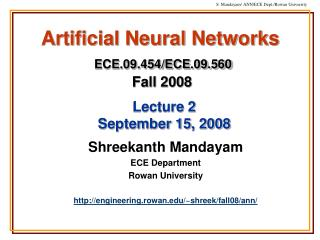DownloadDownload PresentationArtificial Neural Networks ECE.09.454/ECE.09.560 Fall 2008

# Artificial Neural Networks ECE.09.454/ECE.09.560 Fall 2008

Télécharger la présentation## Artificial Neural Networks ECE.09.454/ECE.09.560 Fall 2008

- - - - - - - - - - - - - - - - - - - - - - - - - - - E N D - - - - - - - - - - - - - - - - - - - - - - - - - - -
##### Presentation Transcript

1. Artificial Neural NetworksECE.09.454/ECE.09.560Fall 2008 Lecture 2September 15, 2008 Shreekanth Mandayam ECE Department Rowan University http://engineering.rowan.edu/~shreek/fall08/ann/

2. Plan • Recall: Neural Network Paradigm • Recall: Perceptron Model • Learning Processes • Rules • Paradigms • Tasks • Perceptron Training Algorithm • Widrow-Hoff Rule (LMS Algorithm) • Lab Project 1

3. Indicate Desired Outputs Determine Synaptic Weights Predicted Outputs Recall: Neural Network Paradigm Stage 1: Network Training Artificial Neural Network Present Examples “knowledge” Stage 2: Network Testing Artificial Neural Network New Data

4. Recall: ANN Model x Input Vector y Output Vector Artificial Neural Network f Complex Nonlinear Function “knowledge” f(x) = y

5. Activation/ squashing function wk1 Bias, bk x1 wk2 x2 S S j(.) Output, yk Inputs uk Induced field, vk wkm xm Synaptic weights Recall: The Perceptron Model

6. “Learning” Mathematical Model of the Learning Process Intitialize: Iteration (0) ANN [w]0 x y(0) [w] x y Iteration (1) [w]1 x y(1) desired o/p Iteration (n) [w]n x y(n) = d

7. Learning Rules • Error Correction Learning • Delta Rule or Widrow-Hoff Rule • Memory Based Learning • Nearest Neighbor Rule • Hebbian Learning • Competitive Learning • Boltzman Learning

8. Error-Correction Learning Desired Output, dk (n) wk1(n) Activation/ squashing function x1 (n) Bias, bk wk2(n) x2 + Output, yk (n) S S j(.) Inputs Synaptic weights - Induced field, vk(n) wkm(n) Error Signal ek (n) xm

9. desired Environment (Data) Teacher (Expert) + - ANN S actual error Learning Paradigms Unsupervised Supervised

10. Delay Environment (Data) ANN Cost Function Delayed Reinforcement Learning Learning Paradigms Unsupervised Supervised

11. Pattern Association Pattern Recognition Function Approximation Filtering x2 x2 2 2 DB 1 1 DB x1 x1 Learning Tasks Classification

12. Perceptron Training Widrow-Hoff Rule (LMS Algorithm) w(0) = 0 n = 0 y(n) = sgn [wT(n) x(n)] w(n+1) = w(n) + h[d(n) – y(n)]x(n) n = n+1 Matlab Demo

13. Lab Project 1 • http://engineering.rowan.edu/~shreek/fall08/ann/lab1.html • UCI Machine Learning Repository: • http://www.ics.uci.edu/~mlearn/MLRepository.html • Face Recognition: Generate images

14. Summary